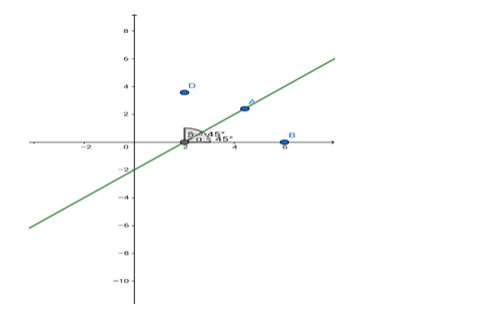# Find the equation of the line cutting off an intercept - 2 from the`
Question:

Find the equation of the line cutting off an intercept - 2 from the y - axis and equally inclined to the axes.

Solution:Given: The line is equally inclined to both the axes.

The angle between the coordinate axes $=90^{\circ}$

If the inclination to both the axes is $\theta$ then $\theta+\theta=90^{\circ}$

i.e. $\theta=45 \theta^{\circ}$

$\therefore$ slope of the line, $m=\tan \theta=\tan 45^{\circ}=1$

The y - intercept = - 2 units

Formula to be used: $y=m x+c$ where $m$ is the slope of the line and $c$ is the $y$ intercept.

The equation of the line is $y=1 \cdot x+(-2)=x-2$

i.e. $x-y=2$